# GeoGebra | Mathematics Centre## GeoGebra

Below you will find some sample applications prepared in Geogebra by Mathematics Center employees

The application can be started by clicking on "run app on GeogebraTube" or directly on the image below its description.

##### Elementary Functions

Sine and cosine functions (run app on GeoGebraTube).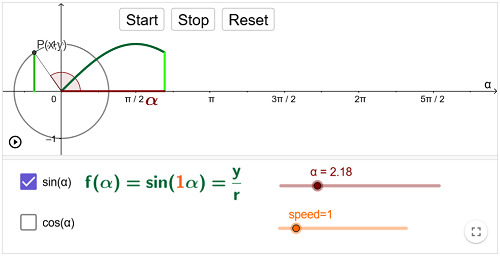Trigonometric and inverse trigonometric functions (run app on GeoGebraTube).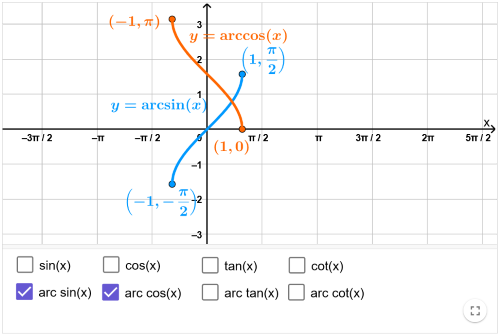##### Number sequences

Limit of a number sequence (run app on GeoGebraTube)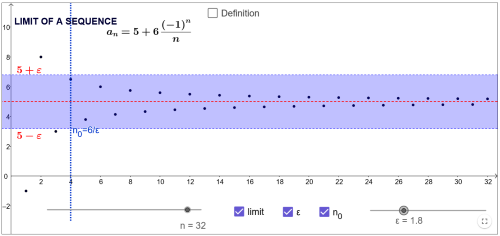##### Limit of a single variable function

Cauchy Definition of limit - examples (run app on GeoGebraTube)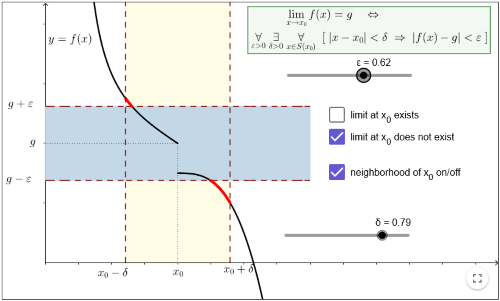##### Derivative of a single variable function

Graphs of functions and their derivatives - examples (run app on GeoGebraTube)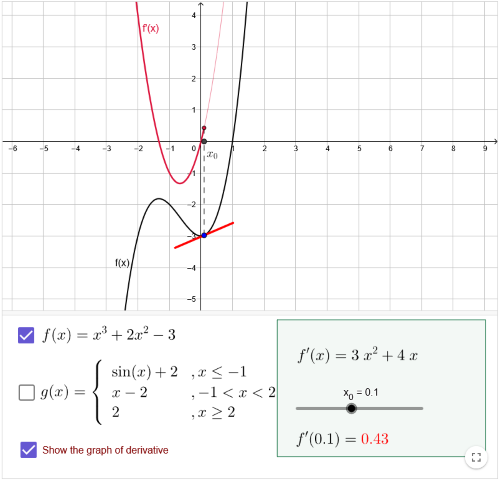##### Improper integral

Improper integral of the first kind - examples (run app on GeoGebraTube)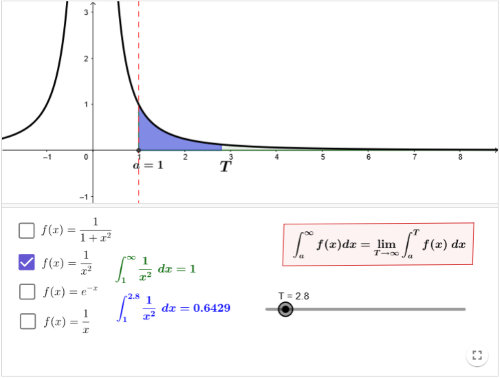Improper integral of the second kind - examples (run app on GeoGebraTube)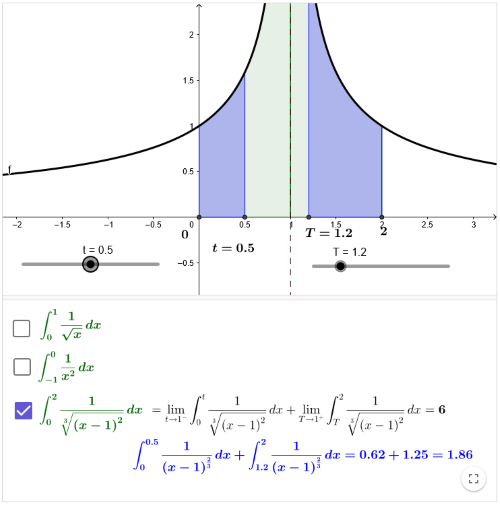##### Change of variables in a tripple integral

Spherical coordinates (run app on GeoGebraTube)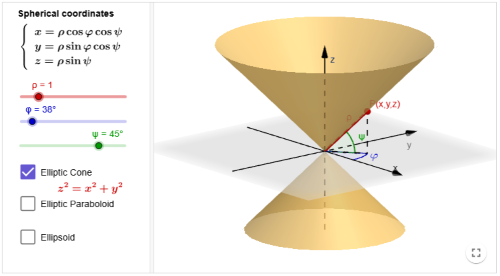##### Number series

Sequence of partial sums - examples (run app on GeoGebraTube)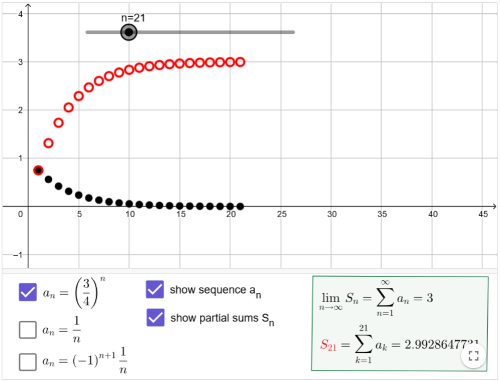##### Fourier Series

Example 1 (run app on GeoGebraTube)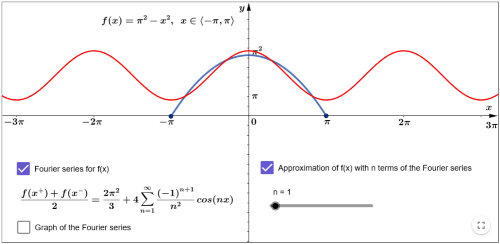Example 2 (run app on GeoGebraTube)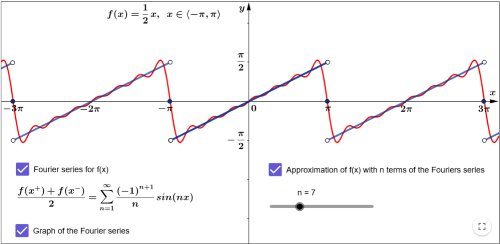##### Complex numbers

Geometrical intepretation of sum and roots of complex numbers (run app on GeoGebraTube)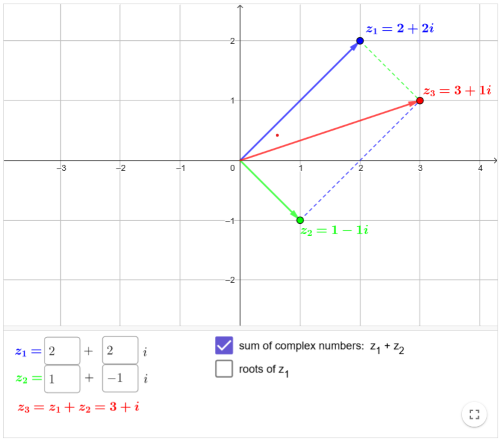Geometrical intepretation of powers of a complex number (run app on GeoGebraTube)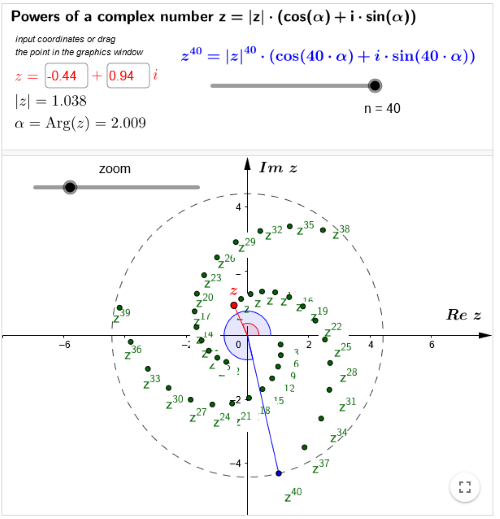Licence CC BY-SA

Mathematics Teaching and Distance Learning Center
Gdańsk University of Technology

## Contact

Content and app manager
dr Anna Niewulis